# Resources tagged with: NC Yr 5

Filter by: Content type:
Age range:
Challenge level:

There are 69 NRICH Mathematical resources connected to NC Yr 5, you may find related items under NC.

Broad Topics > NC > NC Yr 5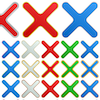### Compare the Calculations

##### Age 7 to 11Challenge Level

Can you put these four calculations into order of difficulty? How did you decide?##### Age 7 to 11Challenge Level

Can you match these calculation methods to their visual representations?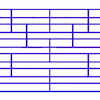### Tumbling Down

##### Age 7 to 11Challenge Level

Watch this animation. What do you see? Can you explain why this happens?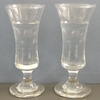### Pouring Problem

##### Age 7 to 11Challenge Level

What do you think is going to happen in this video clip? Are you surprised?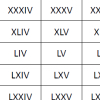### Roman Numerals

##### Age 7 to 11Challenge Level

Can you find some examples when the number of Roman numerals is fewer than the number of Arabic numerals for the same number?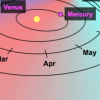### Space Distances

##### Age 7 to 11Challenge Level

This task focuses on distances travelled by the asteroid Florence. It's an opportunity to work with very large numbers.### A4 Fraction Subtraction

##### Age 7 to 11Challenge Level

This task offers opportunities to subtract fractions using A4 paper.##### Age 7 to 11Challenge Level

Try adding fractions using A4 paper.### Subtraction Surprise

##### Age 7 to 14Challenge Level

Try out some calculations. Are you surprised by the results?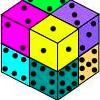### Six Numbered Cubes

##### Age 7 to 11Challenge Level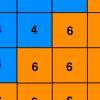### Six Ten Total

##### Age 7 to 11Challenge Level

This challenge combines addition, multiplication, perseverance and even proof.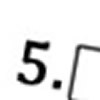### Greater Than or Less Than?

##### Age 7 to 11Challenge Level

Use the numbers and symbols to make this number sentence correct. How many different ways can you find?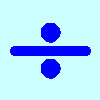### Division Rules

##### Age 7 to 11Challenge Level

This challenge encourages you to explore dividing a three-digit number by a single-digit number.### Multiply Multiples 3

##### Age 7 to 11Challenge Level

Have a go at balancing this equation. Can you find different ways of doing it?### Round the Dice Decimals 2

##### Age 7 to 11Challenge Level

What happens when you round these numbers to the nearest whole number?### Multiply Multiples 2

##### Age 7 to 11Challenge Level

Can you work out some different ways to balance this equation?### Multiply Multiples 1

##### Age 7 to 11Challenge Level

Can you complete this calculation by filling in the missing numbers? In how many different ways can you do it?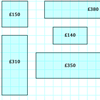### Through the Window

##### Age 7 to 11Challenge Level

My local DIY shop calculates the price of its windows according to the area of glass and the length of frame used. Can you work out how they arrived at these prices?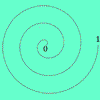### Spiralling Decimals

##### Age 7 to 14Challenge Level

Take turns to place a decimal number on the spiral. Can you get three consecutive numbers?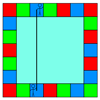### Ribbon Squares

##### Age 7 to 11Challenge Level

What is the largest 'ribbon square' you can make? And the smallest? How many different squares can you make altogether?### Olympic Turns

##### Age 7 to 11Challenge Level

This task looks at the different turns involved in different Olympic sports as a way of exploring the mathematics of turns and angles.### Factor Track

##### Age 7 to 14Challenge Level

Factor track is not a race but a game of skill. The idea is to go round the track in as few moves as possible, keeping to the rules.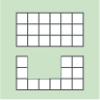### Area and Perimeter

##### Age 7 to 11Challenge Level

What can you say about these shapes? This problem challenges you to create shapes with different areas and perimeters.### Curious Number

##### Age 7 to 11Challenge Level

Can you order the digits from 1-3 to make a number which is divisible by 3 so when the last digit is removed it becomes a 2-figure number divisible by 2, and so on?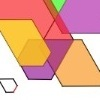### The Numbers Give the Design

##### Age 7 to 11Challenge Level

Make new patterns from simple turning instructions. You can have a go using pencil and paper or with a floor robot.### Three Dice

##### Age 7 to 11Challenge Level

Investigate the sum of the numbers on the top and bottom faces of a line of three dice. What do you notice?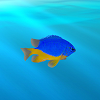### Sea Level

##### Age 7 to 11Challenge Level

The picture shows a lighthouse and many underwater creatures. If you know the markings on the lighthouse are 1m apart, can you work out the distances between some of the different creatures?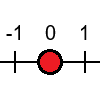### Tug Harder!

##### Age 7 to 11Challenge Level

In this game, you can add, subtract, multiply or divide the numbers on the dice. Which will you do so that you get to the end of the number line first?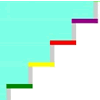### Swimming Pool

##### Age 5 to 11Challenge Level

In this problem, we're investigating the number of steps we would climb up or down to get out of or into the swimming pool. How could you number the steps below the water?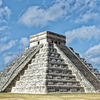### Picture a Pyramid ...

##### Age 7 to 11Challenge Level

Imagine a pyramid which is built in square layers of small cubes. If we number the cubes from the top, starting with 1, can you picture which cubes are directly below this first cube?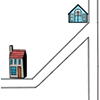### Six Places to Visit

##### Age 7 to 11Challenge Level

Can you describe the journey to each of the six places on these maps? How would you turn at each junction?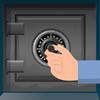### How Safe Are You?

##### Age 7 to 11Challenge Level

How much do you have to turn these dials by in order to unlock the safes?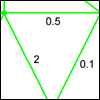### Route Product

##### Age 7 to 11Challenge Level

Find the product of the numbers on the routes from A to B. Which route has the smallest product? Which the largest?### Factors and Multiples Game

##### Age 7 to 16Challenge Level

A game in which players take it in turns to choose a number. Can you block your opponent?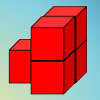##### Age 7 to 11Challenge Level

How can you arrange the 5 cubes so that you need the smallest number of Brush Loads of paint to cover them? Try with other numbers of cubes as well.### More Transformations on a Pegboard

##### Age 7 to 11Challenge Level

Use the interactivity to find all the different right-angled triangles you can make by just moving one corner of the starting triangle.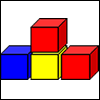### Up and Down Staircases

##### Age 7 to 11Challenge Level

One block is needed to make an up-and-down staircase, with one step up and one step down. How many blocks would be needed to build an up-and-down staircase with 5 steps up and 5 steps down?### Odd Squares

##### Age 7 to 11Challenge Level

Think of a number, square it and subtract your starting number. Is the number you're left with odd or even? How do the images help to explain this?### Trebling

##### Age 7 to 11Challenge Level

Can you replace the letters with numbers? Is there only one solution in each case?### Fitted

##### Age 7 to 11Challenge Level

Nine squares with side lengths 1, 4, 7, 8, 9, 10, 14, 15, and 18 cm can be fitted together to form a rectangle. What are the dimensions of the rectangle?### Which Is Quicker?

##### Age 7 to 11Challenge Level

Which is quicker, counting up to 30 in ones or counting up to 300 in tens? Why?### Transformations on a Pegboard

##### Age 7 to 11Challenge Level

How would you move the bands on the pegboard to alter these shapes?### Matching Fractions, Decimals and Percentages

##### Age 7 to 14Challenge Level

Can you match pairs of fractions, decimals and percentages, and beat your previous scores?### Estimating Angles

##### Age 7 to 14Challenge Level

How good are you at estimating angles?### Cubes Within Cubes

##### Age 7 to 14Challenge Level

We start with one yellow cube and build around it to make a 3x3x3 cube with red cubes. Then we build around that red cube with blue cubes and so on. How many cubes of each colour have we used?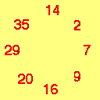### Cycling Squares

##### Age 7 to 11Challenge Level

Can you make a cycle of pairs that add to make a square number using all the numbers in the box below, once and once only?### Two Primes Make One Square

##### Age 7 to 11Challenge Level

Can you make square numbers by adding two prime numbers together?### Multiplication Squares

##### Age 7 to 11Challenge Level

Can you work out the arrangement of the digits in the square so that the given products are correct? The numbers 1 - 9 may be used once and once only.### Reach 100

##### Age 7 to 11Challenge Level

Choose four different digits from 1-9 and put one in each box so that the resulting four two-digit numbers add to a total of 100.### All the Digits

##### Age 7 to 11Challenge Level

This multiplication uses each of the digits 0 - 9 once and once only. Using the information given, can you replace the stars in the calculation with figures?